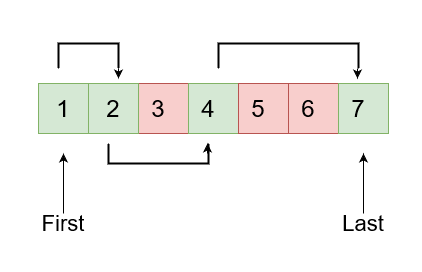New update is available. Click here to update.

# Help Ninja to Cross River

Last Updated: 14 Mar, 2021
Difficulty: Hard

## PROBLEM STATEMENT

#### There are some restrictions on the size of jump Ninja can make:

``````1. The first jump will always be of 1 unit.
2. If Ninja's previous jump was ‘X’ units, his current jump must be either ‘X’ - 1, ‘X’, or ‘X’ + 1 units.
``````

#### For example :

``````If ‘SAFE’ = [1, 2, 4, 7] then it can shown in below image where :
Red cell means that the unit is damaged.
Green cell means that the unit is safe.
We can see Ninja can reach the last unit by making jumps of size 1 , 2 and 3.
``````#### Input Format:

``````The first line of input contains an integer ‘T’ denoting the number of test cases. Then each test case follows.

The first line of each test case contains a single integer ‘N’ denoting the size of ‘SAFE’ or we can say the number of safe units.

The second line contains ‘N’ space-separated distinct integers denoting the elements of ‘SAFE’.
``````

#### Output format:

``````For each test case return, true if Ninja can cross the river else return false.
``````

#### Note :

``````You don’t have to print anything, it has already been taken care of. Just implement the given function.
``````

#### Constraints

``````1 <= T <=10
2 <= N <= 10^3
0 <= SAFE[i] <= 10^5

Time limit: 1 sec
``````## Approach 1

Complete Algorithm:

• First of all, we will make a HashMap say ‘MY_MAP’ to store safe units where the key will be ‘SAFE[i]’ and the value will be ‘i’.
• We will make a helper recursive function named ‘SOLVE’ which will receive 4 parameters ‘SAFE’, ‘MY_MAP’, index of current unit ‘INDEX’ and size of previous jump ‘X’.
• Base Case:
• If ‘INDEX’ is equal to last safe unit:
• Return true.
• Recursive Calls:
• Now we have three possible places to go :
• ‘POS1’ = ‘SAFE[INDEX]’ + ’X’.
• ‘POS2’ = ‘SAFE[INDEX]’ + ‘X’ - 1.
• ‘POS3’ = ‘SAFE[INDEX]’ + ‘X’ +1.
• If “POS1’ exists in ‘MY_MAP’ then do:
• Make a recursive call with ‘INDEX’ as ‘MY_MAP[POS1]’ and keep ‘X’ the same.
• Similarly, we will check for ‘POS2’ and ‘POS3’.
• If the recursive call returns true for any of these three we will return true. The idea is very simple, as standing on any safe unit Ninja will always have a maximum of three choices so we will use recursion to search for all possible options.
• Return the value returned by SOLVE().